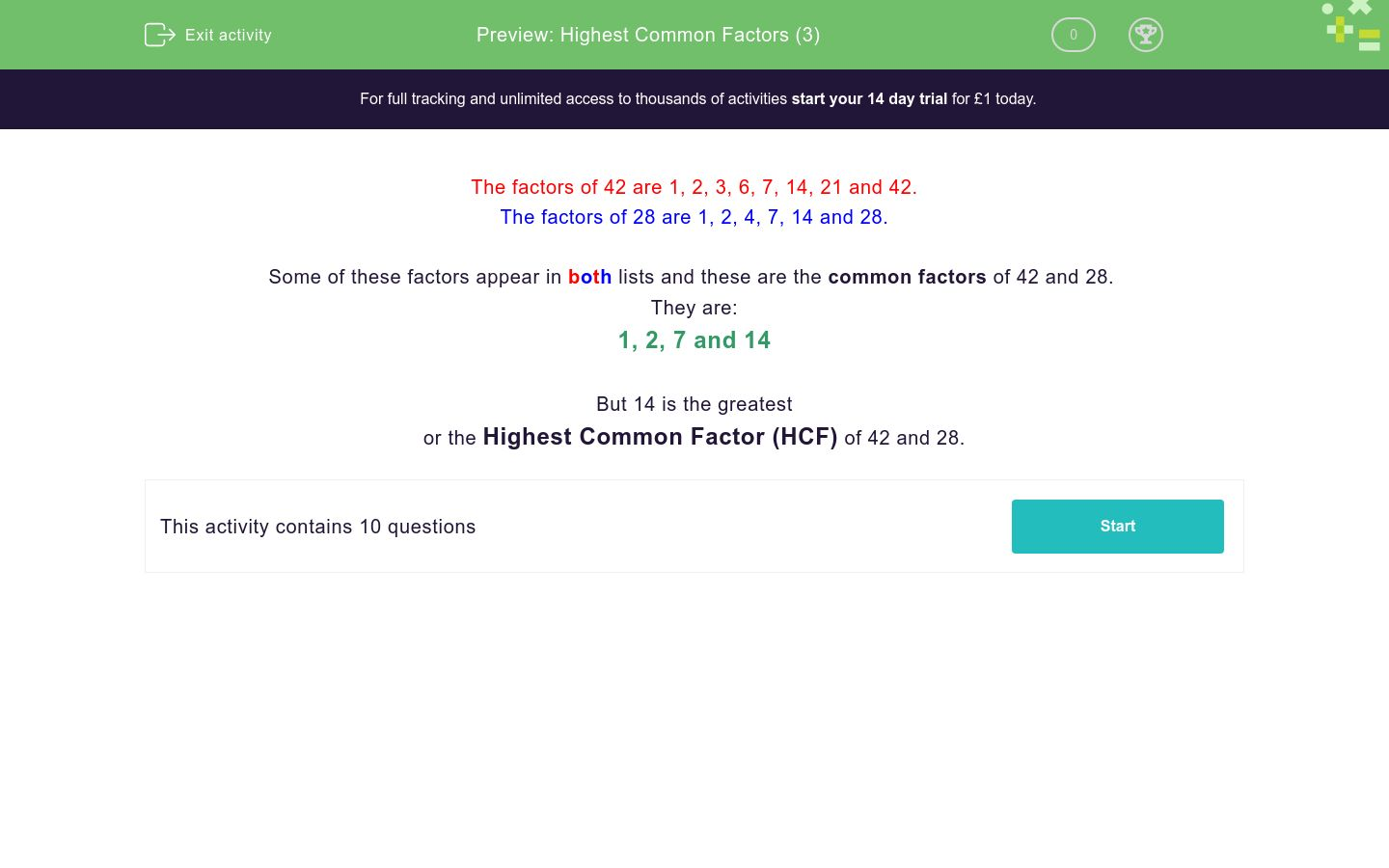# Highest Common Factors (3)

In this worksheet, children must state the highest common factor of two numbers.Key stage:  KS 3

Curriculum topic:  Number

Curriculum subtopic:  Use Concepts and Vocabulary for All Numbers

Difficulty level:### QUESTION 1 of 10

The factors of 42 are 1, 2, 3, 6, 7, 14, 21 and 42.

The factors of 28 are 1, 2, 4, 7, 14 and 28.

Some of these factors appear in both lists and these are the common factors of 42 and 28.

They are:

1, 2, 7 and 14

But 14 is the greatest

or the Highest Common Factor (HCF) of 42 and 28.

What is the highest common factor of....?

42 and 36

What is the highest common factor of....?

60 and 36

What is the highest common factor of....?

30 and 60

What is the highest common factor of....?

28 and 42

What is the highest common factor of....?

31 and 45

What is the highest common factor of....?

16 and 24

What is the highest common factor of....?

42 and 56

What is the highest common factor of....?

63 and 108

What is the highest common factor of....?

48 and 72

What is the highest common factor of....?

69 and 115

• Question 1

What is the highest common factor of....?

42 and 36

6
• Question 2

What is the highest common factor of....?

60 and 36

12
• Question 3

What is the highest common factor of....?

30 and 60

30
• Question 4

What is the highest common factor of....?

28 and 42

14
• Question 5

What is the highest common factor of....?

31 and 45

1
• Question 6

What is the highest common factor of....?

16 and 24

8
• Question 7

What is the highest common factor of....?

42 and 56

14
• Question 8

What is the highest common factor of....?

63 and 108

9
• Question 9

What is the highest common factor of....?

48 and 72

24
• Question 10

What is the highest common factor of....?

69 and 115

23
---- OR ----

Sign up for a £1 trial so you can track and measure your child's progress on this activity.

### What is EdPlace?

We're your National Curriculum aligned online education content provider helping each child succeed in English, maths and science from year 1 to GCSE. With an EdPlace account you’ll be able to track and measure progress, helping each child achieve their best. We build confidence and attainment by personalising each child’s learning at a level that suits them.

Get started#### Similar activities you may like

Start your £1 trial today.
Subscribe from £10/month.